# Arduino SpeedMath Game

SpeedMath Game is a fun way to test your abilities at how fast you can do math in your head both visually and auditorily.

IntermediateWork in progress775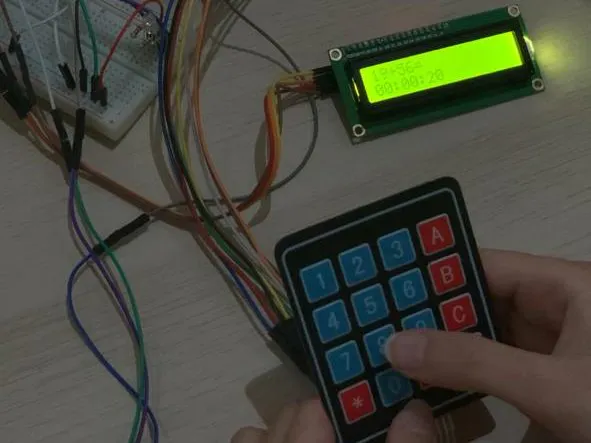## Things used in this project

### Hardware componentsArduino UNO
×1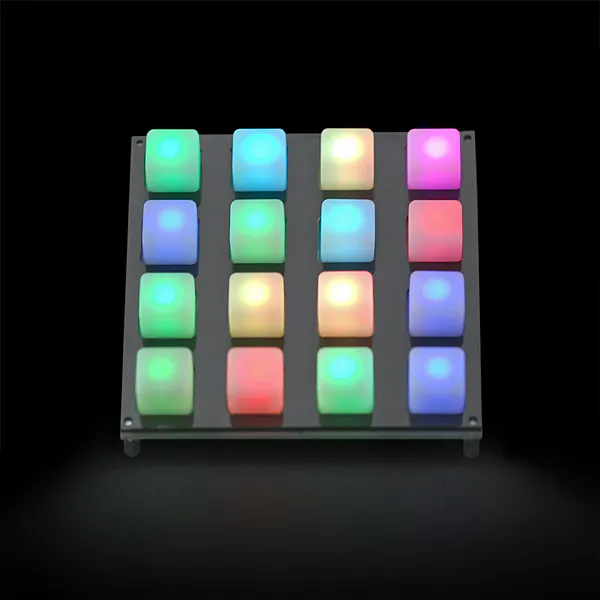SparkFun Button Pad 4x4 - LED Compatible
×1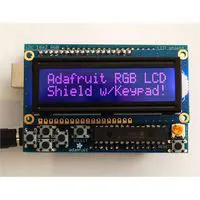RGB LCD Shield Kit, 16x2 Character Display
×1×1
 Infrared IR Obstacle Sensor
×1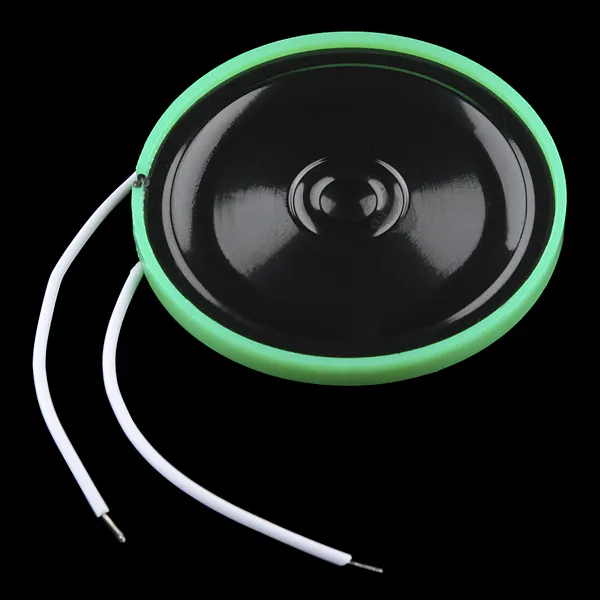Speaker: 0.25W, 8 ohms
×1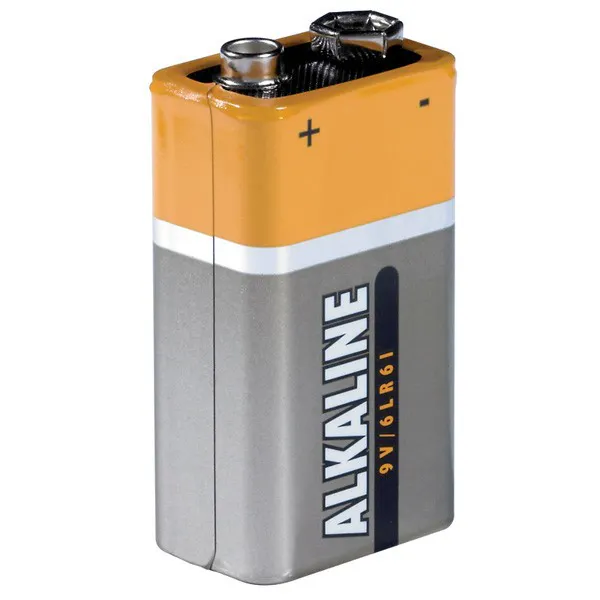9V battery (generic)
×1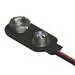9V Battery Clip
×1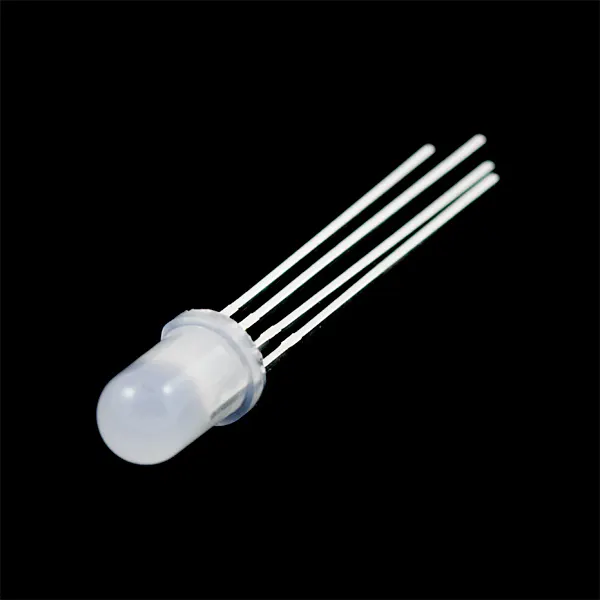RGB Diffused Common Cathode
×1
 Potentiometer 1k Ohms
×1Jumper wires (generic)
×1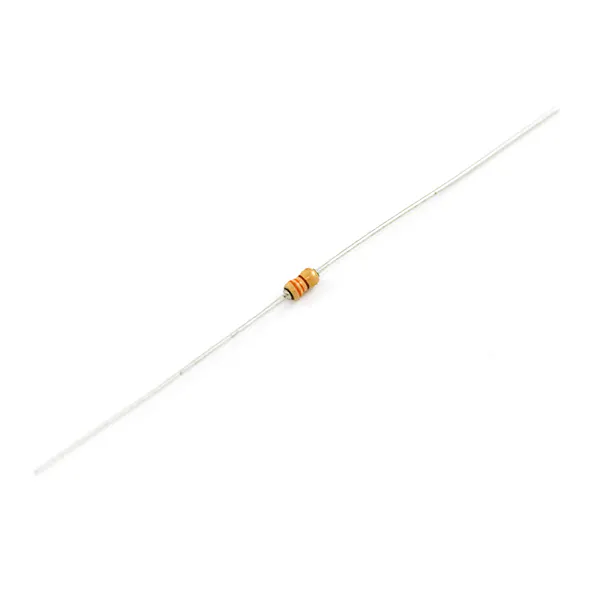Resistor 330 ohm
×3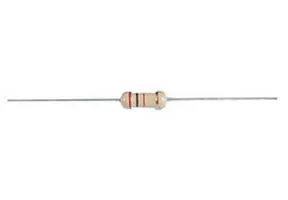Resistor 220 ohm
×1

## Schematics

### Schematic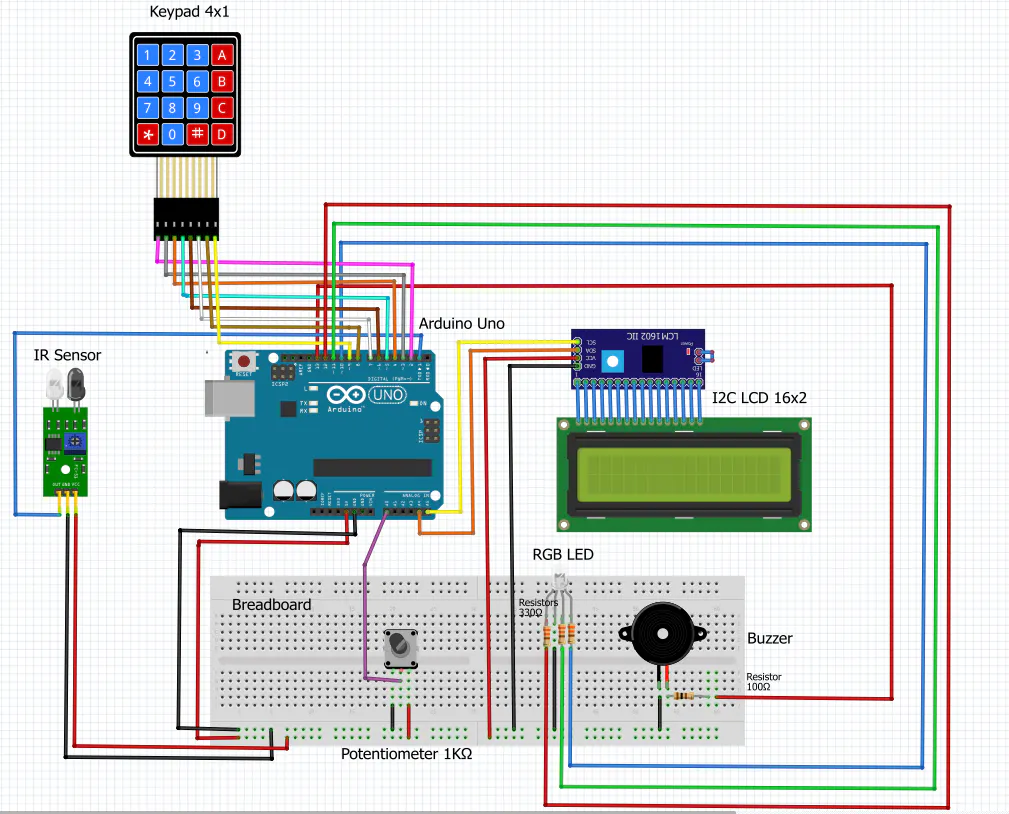## Code

### Code

C/C++
```/* ------------------------------------------------------------------------------------------------------------------------------------------ */
/*                                                         SpeedMath Game                                                                     */
/* ------------------------------------------------------------------------------------------------------------------------------------------ */
/* SpeedMath Game is a fun way to test your abilities at how fast you can do math in your head.                                               */
/* The game contains multiple ways of displaying arithmetic problems, including visually, and auditorily.                                     */
/* ------------------------------------------------------------------------------------------------------------------------------------------ */
/* Instructions:                                                                                                                              */
/* 1. Start the game by waving at the IR sensor. The LED would turn green.                                                                    */
/* 2. The game has 3 levels (Easy, Medium, and Hard).                                                                                         */
/* 3. Click on 1 on the keypad to play the easy level, 2 for the medium level, and 3 for the hard level.                                      */
/* 4. There are 10 questions in each game.                                                                                                    */
/* 5. Make sure to answer quickly, the game is set by a timer for every question. The timer is displayed on the LCD.                          */
/* 6. If you click the wrong key, you can click on "D" on they keypad to erase the last typed number.                                         */
/* 7. To check your answer and move on to the next question before the timer ends, click on "#" on the keypad.                                */
/* 8. If your answer is correct, the green RGB LED lights up. If it is wrong, the LED turns red.                                              */
/* 9. You can adjust the brightness of the LED by turning the knob of the potentiometer.                                                      */
/* 10. If you choose the easy level, you have 20 seconds to answer a "+", "-", "*", or "" math question displayed on the LCD.                */
/* 11. If you choose the medium level, only the operation is displayed on the LCD. You have to observe how many times the blue LED blinks.    */
/* 12. The hard level is similar, except that you have to listen carefully to the speaker and count how many times it "buzzes"!               */
/* 13. For medium and hard levels, a duration of a second separates the first number from the second.                                         */
/* 14. In these two levels, you only have 15 and 10 seconds to perform math operations in your head on the two numbers and type your answers. */
/* 15. At the end of each game, your game score is displayed on the LCD, and then your total game scores.                                     */
/* 16. If you decide to stop the game at any time, you can click on "*" on the keypad.                                                        */
/* 17. Don't worry, you can play the game again at any time by waving at the IR sensor.                                                       */
/* 18. Have fun!                                                                                                                              */
/* ------------------------------------------------------------------------------------------------------------------------------------------ */
/* Made by Mariam Alzaabi                                                                                                                     */
/* 23/11/2020                                                                                                                                 */
/* ------------------------------------------------------------------------------------------------------------------------------------------ */

#include <LiquidCrystal_I2C.h> //LCD library
#include <EEPROM.h> //EEPROM library

// Defining Arduino pins
const byte bluePin = 10; //PB2 attach pin D12 (PB2) Arduino to pin Blue of RGB LED
const byte greenPin = 11; //PB3 attach pin D12 (PB3) Arduino to pin Green of RGB LED
const byte redPin = 12; //PB4 attach pin D12 (PB4) Arduino to pin Red of RGB LED
const byte speaker = 13; //PB5 attach pin D13 (PB5) Arduino to speaker pin
const byte potentiometerPin = A0; //attach pin A0 Arduino to potentiometer pin

// Creating pointers to port D and B registers
byte *ptr_to_PORTD;
byte *ptr_to_PIND;
byte *ptr_to_DDRB;
byte *ptr_to_PORTB;

// New shapes created for the LCD screen
byte smileyFace[] = { //Smiley face used for answers checking
B00000,
B00000,
B01010,
B00000,
B10001,
B01110,
B00000,
B00000
};
byte sadFace[] = { //Sad face used for answers checking
B00000,
B00000,
B01010,
B00000,
B01110,
B10001,
B00000,
B00000
};
byte blankChar[] = { //Blank character used for M/H game levels
B11111,
B11111,
B11111,
B11111,
B11111,
B11111,
B11111,
B11111
};

// Creating object from LCD library to control LCD
LiquidCrystal_I2C lcd(0x27, 16, 2);

const byte ROWS = 4; //four rows
const byte COLS = 4; //four columns

// Defining the keymap
char keys[ROWS][COLS] = {
{'1', '2', '3', 'A'},
{'4', '5', '6', 'B'},
{'7', '8', '9', 'C'},
{'*', '0', '#', 'D'}
};

byte rowPins[ROWS] = {2, 3, 4, 5}; //connect to the row pinouts of the keypad
byte colPins[COLS] = {6, 7, 8, 9}; //connect to the column pinouts of the keypad

// Creating the keypad
Keypad keypad = Keypad( makeKeymap(keys), rowPins, colPins, ROWS, COLS );

// Code for game implementation
namespace Game {
class SpeedMath
{
public:
String input = ""; //used to get a string of number inputs from the user
int num1, num2, correctValue, op; //initialization of int type
String operation = ""; //type of operation used in the game ("+", "-", "*", or "/")
int numChar = 0; //used for determining the number of characters used in typing a question

char difficulty; //level of difficuty chosen by the user (1-E, 2-M, or 3-H)
byte score = 0; //score is initially 0
byte numQuestions = 10; //number of questioned asked, max is 255
bool playMode = false; //if user is in play mode
bool isExited = false; //if the game has already been exited
bool levelChosen = false; //if the level is chosen by the user
bool setUp = false; //if the game has already been exited
bool gameStopped = false; //if the game has been stopped
unsigned long delayStart = 0; //time the delay started
bool delayRunning = false; //true if still waiting for delay to finish
int totScore = 0; //total score of the player
int addTotScore = 0; //address of the total score

// Function used to generate random questions for the easy level game
void generateEasy(void) {
lcd.clear(); //clear LCD screen and position the cursor in the upper-left corner
generateNumbers(1, 100, 1, 100, 1, 20, 1, 100); //the range of randomly generated numbers
lcd.print(String(num1) + operation + String(num2) + "="); //print the question to the LCD
numChar = countDigit(num1) + countDigit(num2) + 2; //count the number of characters displayed on the LCD
delayStart = millis(); //start delay
delayRunning = true; //delay is not finished yet
}

// Function used to generate random questions for the medium level game
void generateMed(void) {
lcd.clear(); //clear LCD screen and position the cursor in the upper-left corner
generateNumbers(1, 10, 1, 10, 1, 10, 1, 10); //the range is [1, 10]
lcd.print("Look carefully!"); //print to the LCD screen for 2 seconds
delay(2000);
lcd.clear(); //clear LCD screen and position the cursor in the upper-left corner
for (int i = 1; i <= num1; i++) { //used for blinking num1
analogWrite(bluePin, 255); //writes an analog value (PWM wave) to blue pin for 250 ms
delay(250);
analogWrite(bluePin, 0); //turn it off for 250 ms
delay(250);
}
delay(1000); //wait for a second before blinking num2
for (int i = 1; i <= num2; i++) { //used for blinking num2
analogWrite(bluePin, 255); //writes an analog value (PWM wave) to blue pin for 250 ms
delay(250);
analogWrite(bluePin, 0); //turn it off for 250 ms
delay(250);
}
delay(1000); //wait for a second before displaying the operation
lcd.createChar(0, blankChar); //create a custom character for displaying the question
lcd.home(); //positions the cursor in the upper-left of the LCD
lcd.write(0); //write the custom character to the LCD
lcd.print(operation); //print the operation to the LCD
lcd.write(0); //write the custom character to the LCD again
lcd.print("="); //print the operation to the LCD
numChar = 4; //number of characters on the LCD
delayStart = millis(); //start delay
delayRunning = true; //delay not finished yet
}

// Function used to generate random questions for the hard level game
void generateHard(void) {
lcd.clear(); //clear LCD screen and position the cursor in the upper-left corner
generateNumbers(1, 10, 1, 10, 1, 10, 1, 10); //the range is [1, 10]
lcd.print("Listen carefully!"); //print to the LCD for 2 seconds
delay(2000);
lcd.clear(); //clear LCD screen and position the cursor in the upper-left corner
for (int i = 1; i <= num1; i++) { //used for "buzzing" num1
tone(speaker, 1000); //start speaker sound for 250ms
delay(250);
noTone(speaker); //Stop buzzing
}
delay(1000); //wait for a second before "buzzing" num2
for (int i = 1; i <= num2; i++) { //used for "buzzing" num1
tone(speaker, 1000); //start speaker sound for 250ms
delay(250);
noTone(speaker); //Stop buzzing
}
delay(1000); //wait for a second before displaying the operation
lcd.createChar(0, blankChar); //create a custom character for displaying the question
lcd.home(); //positions the cursor in the upper-left of the LCD
lcd.write(0); //write the custom character to the LCD
lcd.print(operation); //print the operation to the LCD
lcd.write(0); //write the custom character to the LCD again
lcd.print("="); //print the operation to the LCD
numChar = 4; //number of characters on the LCD
delayStart = millis(); //start delay
delayRunning = true; //delay not finished yet
}

// Function used to reset values after each question
void clean() {
num1 = num2 = correctValue = 0;
input = "";
numChar = 0;
}

// Function used to count the number of digits in a number
int countDigit(int n) {
int count = 0;
while (n != 0) {
n = n / 10;
++count;
}
return count;
}

// Function used to generate the numbers for the game in the range [1, 255]
void generateNumbers(byte minAdd, byte maxAdd, byte minSub, byte maxSub, byte minMul, byte maxMul, byte minDiv, byte maxDiv) {
op = random(1, 5); //generate a random number [1,4] for the type of operation
// Switch statement used for performing mathematical calculation
switch (op) {
case (1): //if the random number is "1", perform addition
operation = "+"; //type of operation is addition
num1 = random(minAdd, maxAdd); //generate a random number [1-99] for the first operand
num2 = random(minAdd, maxAdd); //generate a random number [1-99] for the second operand
correctValue = num1 + num2; //perform addition
break;
case (2): //if the random number is "2", perform subtraction
operation = "-"; //type of operation is subtraction
num1 = random(minSub, maxSub); //generate a random number [1-99] for the first operand
num2 = random(minSub, maxSub); //generate a random number [1-99] for the second operand
while (num1 < num2) { //calculation results cannot be negative
num2 = random(minSub, maxSub);
}
correctValue = num1 - num2; //perform subtraction
break;
case (3): //if the random number is "3", perform multiplication
operation = "x"; //type of operation is multiplication
num1 = random(minMul, maxMul); //generate a random number [1-19] for the first operand
num2 = random(minMul, maxMul); //generate a random number [1-19] for the second operand
correctValue = num1 * num2; //perform multiplication
break;
case (4): //if the random number is "4", perform division
operation = "/"; //type of operation is division
num1 = random(minDiv, maxDiv); //generate a random number [1-99] for the first operand
num2 = random(minDiv, maxDiv); //generate a random number [1-99] for the second operand
while ((num1 < num2) || ((num1 % num2) != 0)) { //calculation results cannot be decimal
num2 = random(minDiv, maxDiv);
}
correctValue = num1 / num2; //perform division
break;
}
}

// Function used for initializing the game
void initGame() {
lcd.clear(); //clear LCD screen and position the cursor in the upper-left corner
int potValue = analogRead(potentiometerPin) / 4; //measure the potentiometer value (max 255)
setColor(0, potValue, 0); //light up the RGB LED with green color
lcd.print("Hello!"); //print to the LCD screen
tone(speaker, 3000, 1000); //start speaker sound for 1 second
delay(1000);
setColor(0, 0, 0); //turn off green color
lcd.clear(); //clear LCD screen and position the cursor in the upper-left corner
lcd.print("Difficulty "); //print to the LCD screen
lcd.setCursor(2, 1); //sLevelet cursor to the third position from the bottom
lcd.print("1-E 2-M 3-H"); //print to the LCD screen
}

// Function used for setting up the game
void setUpGame() {
lcd.backlight(); //turn on blacklight
setUp = true; //the game has been set up
isExited = false; //the game has not been exited
initGame(); //initialize the game
char key;
do { //keep looping until a level is chosen and the user has not chosen to exit the game
key = keypad.getKey(); //value of a key being pressed
if ((key == '1') || (key == '2') || (key == '3')) { //if a level is chosen
delay(1000); //wait for a second before starting
lcd.clear(); //clear LCD screen and position the cursor in the upper-left corner
levelChosen = true; //level has been chosen
difficulty = key; //sets difficulty level
playMode = true; //user is now in play mode
playGame(); //play the game
}
else if (key == '*') { //if user has chosen to stop the game
stopGame(); //stop the game
}
} while (((key != '1') && (key != '2') && (key != '3') && (levelChosen != true)) && (!isExited));
}

// Function for playing the game based on difficulty level
void playGame() {
numQuestions -= 1; //decrement number of questions
// Switch statement used for generating questions based on difficulty level
switch (difficulty) {
case ('1'): //if difficulty is easy
generateEasy(); //generate random easy questions
break;
case ('2'): //if difficulty is medium
generateMed(); //generate random medium questions
break;
case ('3'): //if difficulty is hard
generateHard(); //generate random hard questions
break;
}
}

// Function used to check if the inputted values match the correct values
lcd.clear(); //clear LCD screen and position the cursor in the upper-left corner
int potValue = analogRead(potentiometerPin) / 4; //measure the potentiometer value (max 255)
if (input.toInt() == correctValue) { //if they match
score += 1; //increment the score value
tone(speaker, 4500, 800); //start speaker sound for 1 second
lcd.createChar(0, smileyFace); //create a custom character (smiley face)
lcd.home(); //positions the cursor in the upper-left of the LCD
lcd.print("Correct!"); //print to the LCD screen
lcd.write(0); //write the custom character to the LCD
setColor(0, potValue, 0); //light up the RGB LED with green color
} else { //if they do not match
tone(speaker, 500, 800); //start speaker sound for 1 second
lcd.createChar(0, sadFace); //create a custom character (sad face)
lcd.home(); //positions the cursor in the upper-left of the LCD
lcd.print("Incorrect!"); //print to the LCD screen
lcd.write(0); //write the custom character to the LCD
setColor(potValue, 0, 0); //light up the RGB LED with red color
}
delay(1000); //wait for 1 second
setColor(0, 0, 0); //turn off RGB LED color
lcd.clear(); //clear LCD screen and position the cursor in the upper-left corner
clean(); //reset values
}

//Function to check if there are questions left
void continueGame() {
if (numQuestions > 0) { //if there are questions left
playGame(); //keep playing the game
} else if (numQuestions == 0 && playMode == true) { //if no questions left
playMode = false; //user has finished the game
lcd.setCursor(5, 0); //set cursor to the sixth position from the top
lcd.print("Score:" + String(score) + "/10"); //print to the LCD screen
delay(1500); //wait for 1.5 seconds
lcd.clear(); //clear LCD screen and position the cursor in the upper-left corner
lcd.print("Total Score"); //print to the LCD screen
lcd.setCursor(0, 1); //set cursor to the first position from the bottom
totScore = EEPROM.get(addTotScore, totScore) + score; //update total score
EEPROM.put(addTotScore, totScore); //write total score to the EEPROM
lcd.print(String(EEPROM.get(addTotScore, totScore))); //print total score on the LCD from EEPROM
}
}

// Function for stopping the game
void stopGame() {
if (!isExited) { //stop the game if it has not been already stopped
clean(); //reset values
lcd.clear(); //clear LCD screen and position the cursor in the upper-left corner
setColor(255, 0, 0); //light up the RGB LED with red color
lcd.setCursor(6, 0); //set cursor to the seventh position from the top
lcd.print("Good"); //print to the LCD screen
lcd.setCursor(6, 1); //set cursor to the seventh position from the bottom
lcd.print("Bye!"); //print to the LCD screen
delay(2000); //clear everything after 2 seconds
setColor(0, 0, 0); //turn off red color
lcd.clear(); //clear LCD screen and position the cursor in the upper-left corner
clearGame(); //reset values
}
}

// Function for resetting values after every game
void clearGame() {
lcd.noBacklight(); //turn off backlight
isExited = true; //the game has been stopped
setUp = false; //the game can be set up when it is on again
numQuestions = 10; //the number of questions is back to 10
difficulty = NULL; //difficulty can be chosen again later
levelChosen = false; //level can be chosen again later
score = 0; //reset the score
}

// Function used when a number is pressed on the Keypad
void numPress(char num) {
if (playMode) { //if the user is in play mode
lcd.setCursor(numChar, 0); //set the cursor to the position after the printed characters
input += num; //convert the numbers to a string of numbers
numChar += 1; //increment the number of characters displayed on the LCD
lcd.write(num); //write the number to the LCD
tone(speaker, 1000, 300); //start speaker sound for 1 second
}
}

// Function used to delete a character typed during the game
void deleteChar() {
if (input.length() != 0) { //if there are characters typed by the user
numChar -= 1; //decrement the number of characters displayed on the LCD
input.remove(input.length() - 1); //remove the last character from the input string
lcd.setCursor(numChar, 0); //set the cursor to the last position
lcd.print(" "); //hide the character from the LCD
lcd.setCursor(numChar, 0); //set the cursor to the last position
}
}

// Use PWM to control the brightness of the RGB LED [0-255]
void setColor(int redValue, int greenValue, int blueValue) {
analogWrite(redPin, redValue); //writes an analog value (PWM wave) to red pin
analogWrite(greenPin, greenValue); //writes an analog value (PWM wave) to green pin
analogWrite(bluePin, blueValue); //writes an analog value (PWM wave) to blue pin
}

//Function used to set up the timer based on difficulty level
void setUpTimer() {
// Switch statement used for setting the timer based on difficulty level
switch (difficulty) {
case ('1'): //if difficulty is easy
setTimer(20); //sets a timer of 20 seconds
break;
case ('2'): //if difficulty is medium
setTimer(15); //sets a timer of 10 seconds
break;
case ('3'): //if difficulty is hard
setTimer(10); //sets a timer of 10 seconds
break;
}
}

//Function used to create the timer
void setTimer(unsigned long inSeconds) {
if (playMode) { //if the game is in play mode
if (inSeconds < 86400) { //maximum is 23h:59m:59s = 86399 seconds
bool secondPrinted = false; //can only be printed once
// Check if delay has timed out after 5 seconds
if (delayRunning && ((millis() - delayStart) >= (inSeconds * 1000))) {
delayRunning = false; //this code can only run once
continueGame(); //continue playing the game
}
else { //if the timer has not timed out
for (int i = 1; i <= inSeconds; i++) { //check if another second has passed
if ((delayRunning && ((millis() - delayStart) >= ((inSeconds - i) * 1000))) && !secondPrinted) {
secondPrinted = true; //only check for the first correct condition
displayTimer(i); //display the timer on the LCD
}
}
}
}
}
}

//Function used to display the timer on the LCD
void displayTimer(int totalSecond) {
lcd.setCursor(0, 1); //set the cursor to the first position from the bottom
char timeFormat; //for formatting the time

//Return a formatted string in the form HH:MM:SS
sprintf(timeFormat, "%02d:%02d:%02d", totalSecond / 3600, (totalSecond % 3600) / 60, totalSecond % 60);
lcd.print(timeFormat); //print it to the LCD
}
};
}

// Initializing an object of class SpeedMath
Game::SpeedMath my_game;

// Setup code here, to run once
void setup() {
lcd.init(); //initialize the LCD
ptr_to_PORTD = 0x2B; //PORTD (port D) register address
ptr_to_PIND = 0x29; //PIND (port D) register address
*ptr_to_PORTD = B00000010; //sets IR pin in PD1 as input
ptr_to_DDRB = 0x24; //DDRB (port B) register address
ptr_to_PORTB = 0x25; //PORTB (port B) register address
*ptr_to_DDRB = B00111100; //sets speaker pin in PB5 and RGB pins in PB2/3/4 as output
randomSeed(analogRead(0)); //seeds the random number generator (AnalogRead on pin 0)
}

// Main code, to run repeatedly
void loop() {
// Set up a timer based on difficulty level
my_game.setUpTimer();
byte statusIRSensor = *ptr_to_PIND; //digitalRead(IRSensor);
if (!my_game.setUp && ((statusIRSensor & B00000010) == LOW)) //if the game has not been set up and an object has been detected
{
my_game.setUpGame(); //set up the game
}
char key = keypad.getKey(); //value of a key being pressed
if (key) { //if a key has been pressed
// Switch statement used when a key is pressed on the Keypad
switch (key) {
case 'A': //if any of these are pressed nothing happens
case 'B':
case 'C':
break;
case 'D': //if 'D' is pressed
my_game.deleteChar(); //delete the last character typed
break;
case '#': //if '#' is pressed
my_game.continueGame(); //check the answer then continue the game if there are questions left
break;
case '*': //if '*' is pressed
my_game.stopGame(); //stop the game
break;
default: //if a number is pressed
my_game.numPress(key); //write the number to the LCD and retrieve a string of the numbers pressed
break;
}
}
}
```